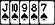Select Page
Poker Forum
Over 1,287,000 Posts!
Poker ForumFTR Community

1.  10-24-2020 01:00 PMMadMojoMonkeyAINT SHOVELING SHITJoin Date Apr 2012 Posts 9,546 Location St Louis, MO P dV is mechanical work. Work is energy, as the Work/Energy Theorem reminds us. Both have units of Joules. [digression] So does torque, which is a little confusing, but also interesting. 1 Nm = 1 J. It results from radians being a dimensionless unit, so angular velocity squared has the same units as angular acceleration, which is not true for linear terms. Defining radians: radius*{radians} = arc length {radians} = {arc length} / {radius} Both arc length and radius are measured in length, so the units of radians vanish: [radian] = [m/m] = 1 So the angular velocity, squared has the same units as the angular acceleration: [rad/s]^2 = [rad/s^2] but this is not true for linear motion, because the numerator has non-vanishing units. [m/s]^2 != [m/s^2] Square brackets indicate I'm only talking about the units. [/digression] Like the pushing of a piston in a car engine. There's an increase in pressure due to the combustion, the piston moves, changing the volume, and the work (energy) transmitted to the piston is P(x) * A dx, since the area of the piston head is constant, and the changing volume is due to movement in 1-dimension. Here, P(x) is the pressure as a function of x, and A is the surface area of the top of the piston, dx is the distance traveled by the piston, due to the volume of the cylinder changing by a factor of ~7 in a gasoline engine, the pressure change is not negligible. (The volume change is only more dramatic in diesel engines, which initiate combustion only by compression of the fuel-air mixture, without the injection of heat cause by a spark, so they need to compress the fuel-air more to achieve the heat needed for spontaneous combustion.) You can find any pattern you want to any level of precision you want, if you're prepared to ignore enough data.Reply With Quote